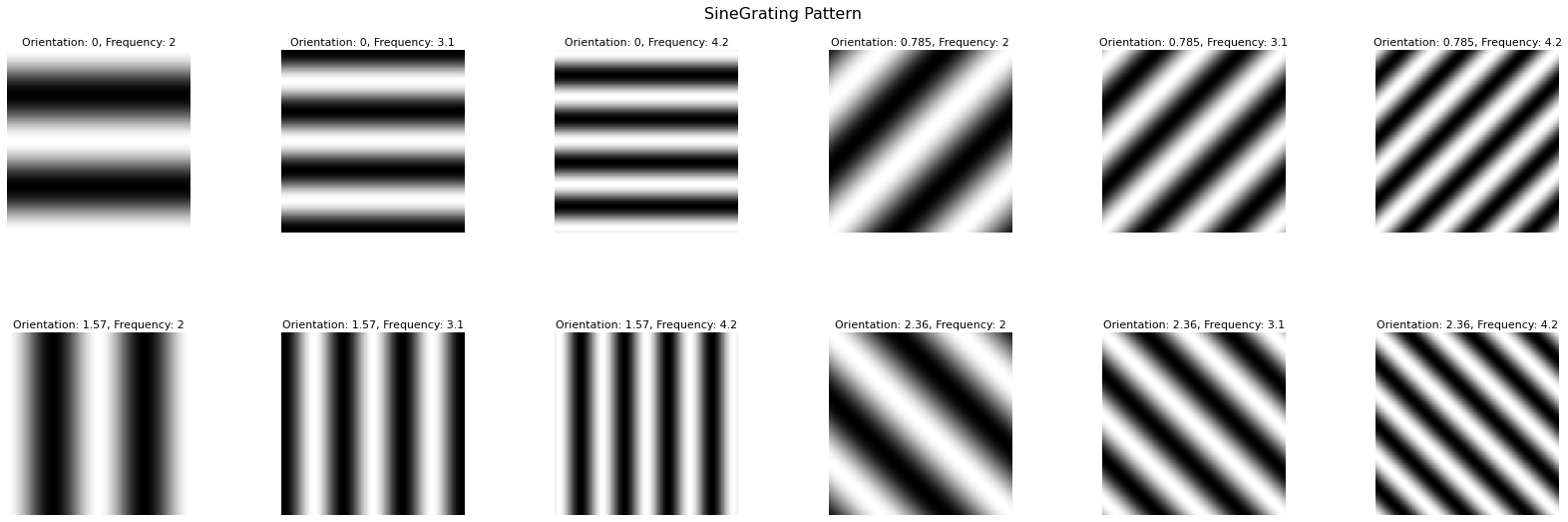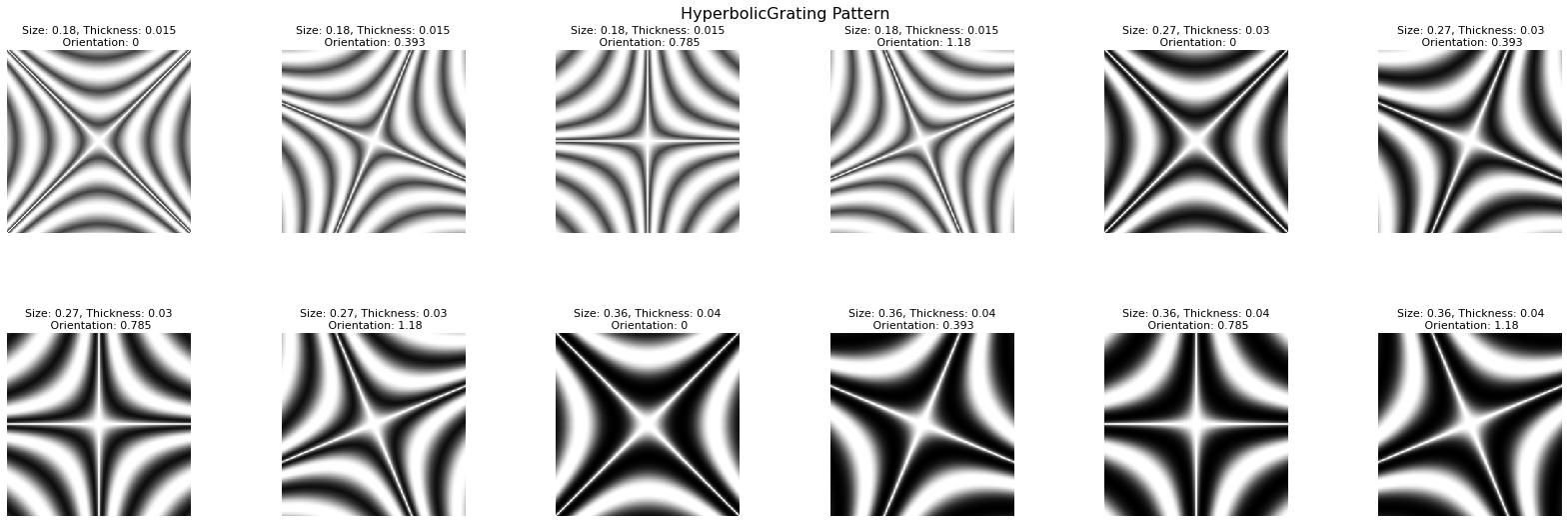# hegde cc07¶

## Visual patterns from Hegde and Van Essen (2007)¶

Visual test patterns implemented by Jiri Machalek and James A. Bednar from:

J. Hegde and D. Van Essen, A comparative study of shape representation in macaque visual areas V2 and V4, Cerebral Cortex (2007) 17:1100-1116. http://dx.doi.org/10.1093/cercor/bhl020

Includes various grating and contour stimuli subclasses. Stimuli from one subclass have common shape characteristics but vary in orientation, size and/or spatial frequency. Patterns have not been matched bit for bit to the originals, but should be reasonably equivalent.

In :
import imagen as ig
import holoviews as hv
import numpy as np

In :
%output dpi=150 size=100
%opts Layout [sublabel_format="" vertical_spacing=0.05 horizontal_spacing=0.05] Image (cmap='gray') [show_xaxis=None show_yaxis=None show_frame=True]
from imagen import *
variants = 4 # Number of variants for each subclass

In :
orientations = (i*np.pi/variants for i in range(variants))
frequencies = [2.0, 3.1, 4.2]
sin = {(o, f): SineGrating(phase=np.pi/2, frequency=f,orientation=o)
for o in orientations for f in frequencies}
sinusoidal = hv.NdLayout({k:v[:] for k,v in sin.items()}, key_dimensions=['Orientation', 'Frequency'])
sinusoidal.cols(6)

Out:In :
size_thickness = zip([0.18, 0.27, 0.36], [0.015, 0.03, 0.04])
orientations = (i*np.pi/(2*variants) for i in range(variants))
hyp = {(s, t, o): HyperbolicGrating(size=s, thickness=t, orientation=o)
for o in orientations for (s, t) in size_thickness}
hyperbolic = hv.NdLayout({k:v[:] for k,v in hyp.items()}, key_dimensions=['Size', 'Thickness', 'Orientation'])
hyperbolic.cols(6)

Out:In :
pol1 = [ConcentricRings(size=0.35/(1+j*0.5),thickness=0.05/(1+j*0.35),smoothing=0.05/(1+j*0.15)) for j in range(variants)]

pol2 = [SpiralGrating(parts=(j+1)*2,turning=0.19+0.30*j,smoothing=0.110+0.015*j) for j in range(variants)]
pol3 = [SpiralGrating(parts=(j+1)*2,turning=0.09+0.13*j,smoothing=0.050+0.006*j) for j in range(variants)]
pol4 = [SpiralGrating(parts=(j+1)*2,turning=0.06+0.09*j,smoothing=0.035+0.006*j) for j in range(variants)]
pol5 = [SpiralGrating(parts=(j+1)*2,turning=0.05+0.07*j,smoothing=0.030+0.003*j) for j in range(variants)]

pol6 = [RadialGrating(parts=(j+1)*2) for j in range(variants)]

polar = pol1 + pol2 + pol3 + pol4 + pol5 + pol6

np.sum([p[:] for p in polar]).cols(8)

Out: